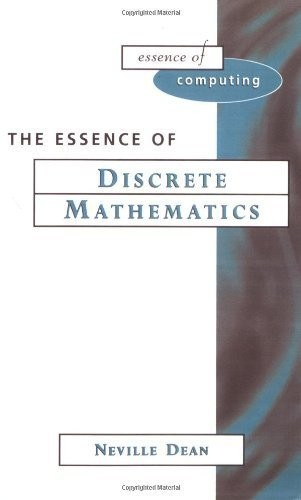# Essence of Discrete Mathematics by Neville Dean

This book is appropriate for a first course in Discrete Mathematics. The fundamentals of sets and logic supply the foundations for learning and provide clear instructions on how to calculate values for mathematical expressions for small finite sets.

5 in stock

## Essence of Discrete Mathematics Summary

The Prentice Hall Essence of Computing Series provides a concise, practical and uniform introduction to the core components of an undergraduate computer science degree. Acknowledging recent changes within Higher Education, this approach uses a variety of pedagogical tools-case studies, worked examples and self-test questions to underpin the student's learning.<br /><br />The essence of Discrete Mathematics provides under one cover all the essential topics covered in a first course on discrete mathematics. Following an introductory chapter, which explains to the reader how to use the book, there follows chapters on sets and logic. In these chapters every effort has been made to give the reader clear instructions on how to 'calculate' values for mathematical expressions for small finite sets. Since it is best to use examples familiar to the reader, sets of numbers are used extensively. Nevertheless it is important for the reader to examine non-numerical examples, and so a case study is introduced at the end of the chapter on sets and then subsequently used throughout the remainder of the book. <br /><br />Once these basic skills have been mastered, the reader progresses on to relations and functions. These are first introduced as intuitive notions before explaining how they can be modelled using sets. There follows a chapter showing how the ideas of modelling with sets and logic can be applied to more practical problems. Finally there is a brief concluding chapter which invites readers to continue their mathematical growth: The Essence of Discrete Mathematics is meant to be the beginning and not the end.

### Why buy from World of BooksOur excellent value books literally don't cost the earthFree delivery in the UKEvery used book bought is one saved from landfill

GOR001219049
Essence of Discrete Mathematics
Neville Dean
Used - Very Good
Paperback
Pearson Education (US)
1996-08-20
208
0133459438
9780133459432
N/A
Book picture is for illustrative purposes only, actual binding, cover or edition may vary.
This is a used book - there is no escaping the fact it has been read by someone else and it will show signs of wear and previous use. Overall we expect it to be in very good condition, but if you are not entirely satisfied please get in touch with us.